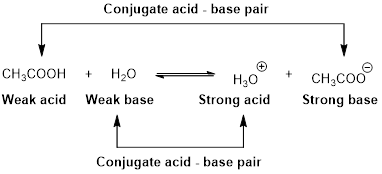# Write the formula and the give the name of the conjugate base of each of the following acids. a) HCN b) HSO 4 − c) HF### Chemistry & Chemical Reactivity

9th Edition
John C. Kotz + 3 others
Publisher: Cengage Learning
ISBN: 9781133949640### Chemistry & Chemical Reactivity

9th Edition
John C. Kotz + 3 others
Publisher: Cengage Learning
ISBN: 9781133949640

#### Solutions

Chapter 16, Problem 1PS
Textbook Problem

## Write the formula and the give the name of the conjugate base of each of the following acids. a) HCN b) HSO 4 − c) HF

Expert Solution

(a)

Interpretation Introduction

Interpretation:

Formula and the conjugate base of given acid has to be written.

Concept Introduction:

Arrhenius Acids and Bases

Acid release hydrogen ion in water

Base release hydroxide ions in water.

HCl(aq) H+(aq) + Cl-(aq).........AcidNaOH(aq) Na+(aq)+ OH-(aq)...........Base

An acid is a substance that produces hydronium ions, H3O+ when dissolved in water.

Bronsted –Lowry definitions:

A Bronsted –Lowry acid is a proton donor, it donates a hydrogen ion, H+

A Bronsted-Lowry base is a proton acceptor, it accepts a hydrogen ion H+.Lewis definition:

A Lewis acid is a substance that can accept and share an electron pair.

A Lewis base is a substance that can donate and share an electron pair.Bronsted –Lowry conjugate acid-Base pairs:

When an acid is dissolved in water, the acid (HA) donates a proton to water to form a new acid (conjugate acid) and a new base (conjugate base).The conjugate base of HCN is Cyanide ion (CN-)

### Explanation of Solution

HCN is an acid .Cyanide ion (CN-) is a conjugate base. HCN and (CN-) is differs by only one proton. Therefore, (CN-) is a conjugate base of HCN

Expert Solution

(b)

Interpretation Introduction

Interpretation:

Formula and the conjugate base of given acid has to be written.

Concept Introduction:

Arrhenius Acids and Bases

Acid release hydrogen ion in water

Base release hydroxide ions in water.

HCl(aq) H+(aq) + Cl-(aq).........AcidNaOH(aq) Na+(aq)+ OH-(aq)...........Base

An acid is a substance that produces hydronium ions, H3O+ when dissolved in water.

Bronsted –Lowry definitions:

A Bronsted –Lowry acid is a proton donor, it donates a hydrogen ion, H+

A Bronsted-Lowry base is a proton acceptor, it accepts a hydrogen ion H+.Lewis definition:

A Lewis acid is a substance that can accept and share an electron pair.

A Lewis base is a substance that can donate and share an electron pair.Bronsted –Lowry conjugate acid-Base pairs:

When an acid is dissolved in water, the acid (HA) donates a proton to water to form a new acid (conjugate acid) and a new base (conjugate base).The conjugate base of HSO4- is sulphate ion SO42-.

### Explanation of Solution

HSO4- is an acid .sulphate ion (SO42-) is a conjugate base. HSO4- and (SO42-) is differs by only one proton. Therefore,( SO42-) ion is a conjugate base of HSO4-

Expert Solution

(c)

Interpretation Introduction

Interpretation:

Formula and the conjugate base of given acid has to be written.

Concept Introduction:

Arrhenius Acids and Bases

Acid release hydrogen ion in water

Base release hydroxide ions in water.

HCl(aq) H+(aq) + Cl-(aq).........AcidNaOH(aq) Na+(aq)+ OH-(aq)...........Base

An acid is a substance that produces hydronium ions, H3O+ when dissolved in water.

Bronsted –Lowry definitions:

A Bronsted –Lowry acid is a proton donor, it donates a hydrogen ion, H+

A Bronsted-Lowry base is a proton acceptor, it accepts a hydrogen ion H+.Lewis definition:

A Lewis acid is a substance that can accept and share an electron pair.

A Lewis base is a substance that can donate and share an electron pair.Bronsted –Lowry conjugate acid-Base pairs:

When an acid is dissolved in water, the acid (HA) donates a proton to water to form a new acid (conjugate acid) and a new base (conjugate base).The conjugate base of HF is fluoride ion (F).

### Explanation of Solution

HF is an acid .Fluoride ion (F-) is a conjugate base. HF and (F-) is differs by only one proton. Therefore, Fluoride (F-) is a conjugate base of HF

### Want to see more full solutions like this?

Subscribe now to access step-by-step solutions to millions of textbook problems written by subject matter experts!

### Want to see more full solutions like this?

Subscribe now to access step-by-step solutions to millions of textbook problems written by subject matter experts!